# Gaussian Surface

## What is Gaussian Surface?

The Gaussian surface is known as a closed surface in three-dimensional space such that the flux of a vector field is calculated. These vector fields can either be the gravitational field or the electric field or the magnetic field. Using Gauss law, Gaussian surface can be calculated: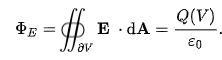Where Q(V) is the electric charge contained in the V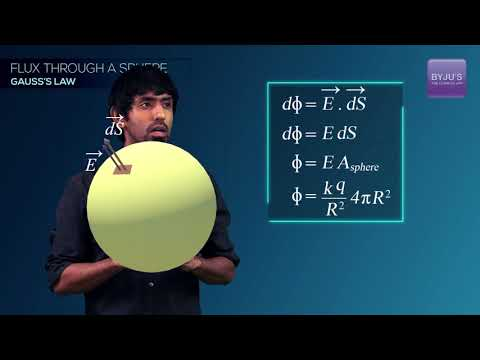## Gaussian Surface of a Sphere

When a flux or electric field is produced on the surface of the spherical Gaussian surface due to any of the following:

• A point charge
• A spherical shell with the uniform charge distribution
• Charge distribution with spherical symmetry

Consider a spherical shell S with uniform distribution of charge Q, radius R and with negligible thickness. Using Gauss law to find electric field E at a distance r from the centre of the charged shell. As r < R, net flux and the magnitude of the electric field on the Gaussian surface are zero.

Following is the flux out of the spherical surface S with surface area of radius r is given as: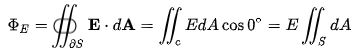$$\iint_{S}dA=4\pi r^{2}$$ $$\Phi _{E}=\frac{Q_{A}}{\epsilon _{0}}$$ (flux by Gauss law)

$$E4\pi r^{2}=\frac{Q_{A}}{\epsilon _{0}}\Rightarrow E=\frac{Q_{A}}{4\pi \epsilon _{0}r^{2}}$$

The above equation shows the spherical distribution of charge which acts as a point charge verifying Coulomb’s law.

You may also want to check out these topics given below!

## Gaussian Surface of Cylinder

When a flux or electric field is produced on the surface of a cylindrical Gaussian surface due to any of the following:

• Uniform distribution of charge in an infinitely long line
• Uniform distribution of charge in an infinite plane
• Uniform distribution of charge on an infinitely long cylinder

Consider a point charge P at a distance r having charge density λ of an infinite line charge. The axis of rotation for the cylinder of length h is the line charge, following is the charge q enclosed in the cylinder:

 $$q=\lambda h$$

Following is the flux out of the cylindrical surface with the differential vector area dA on surfaces a, b and c are given as: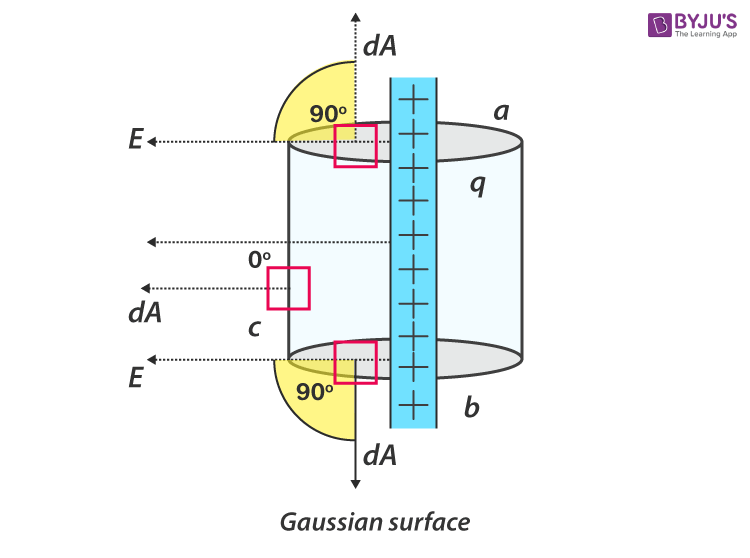Gaussian Surface of Cylinder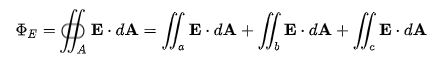$$\Phi_{E}=\iint_{a}EdAcos90^{\circ}+\iint_{b}EdAcos90^{\circ}+\iint_{c}EdAcos0^{\circ}$$ $$=E\iint_{c}dA$$ $$\iint_{c}dA=2\pi rh$$ (which is the surface area of the cylinder)

$$\Phi _{E}=E2\pi rh$$ $$\Phi _{E}=\frac{q}{\epsilon _{0}}$$ (by Gauss law)

$$E2\pi rh=\frac{\lambda h}{\epsilon _{0}}\Rightarrow E=\frac{\lambda }{2\pi \epsilon _{0}r}$$

The above equation shows the cylindrical Gaussian surface with a uniform distribution of charges.## Gaussian Surface Equations

 The Gaussian surface of a sphere $$E=\frac{Q_{A}}{4\pi \epsilon _{0}r^{2}}$$ The Gaussian surface of a cylinder $$E=\frac{\lambda }{2\pi \epsilon _{0}r}$$

### What is Gaussian Pillbox?

The Gaussian pillbox is the surface with an infinite charge of uniform charge density is used to determine the electric field. The pillbox is of a cylindrical shape consisting of three components; the disk at one end with area 𝝿r4, the disk at the other end with the equal area and the side of the cylinder. As stated by Gauss law, the sum of electric flux through each component is proportional to the enclosed charge of the pillbox.

### Gauss Law ExplainedStay tuned with BYJU’S for more such interesting articles. Also, register to “BYJU’S – The Learning App” for loads of interactive, engaging Physics-related videos and an unlimited academic assist.

Test your knowledge on Gaussian Surface